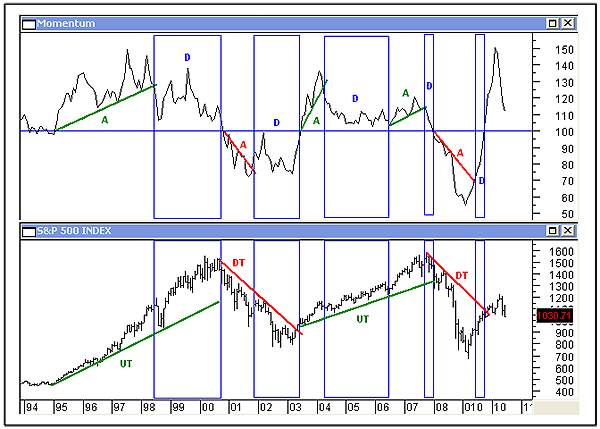HOT TOPICS LIST

INDICATORS LIST

LIST OF TOPICS

# Momentum Indicator Measures Momentum

07/07/10 09:00:20 AM
by Alan R. Northam

It is not the acceleration period of momentum that is important, it is the deceleration period.

Security:   SPX
Position:   N/A

 Momentum indicators measure the rate of the rise and fall of stock prices. When the momentum indicator is rising, it indicates that the price of the stock is accelerating, and when the momentum indicator is falling, it indicates that the price of the stock is decelerating. There are many different momentum indicators that a trader can use, but they all measure just two things; acceleration and deceleration of price. The momentum indicator discussed here is the simplest of the momentum indicators. The indicator is calculated by dividing the current closing price by the closing price of some lookback period. The longer the lookback period, the longer the momentum of the trend that is being measured. The lookback period for the momentum indicator plotted in Figure 1 is 12 months. Therefore, this chart is measuring the long-term momentum of the Standard & Poor's 500.Figure 1 is the monthly chart of the S&P 500. It contains two charts: the momentum chart above and the price chart below. On the price chart I have identified two uptrends and two downtrends. The uptrends are highlighted in green and labeled as "UT" for upward trend. The downtrends are highlighted in red and labeled "DT" for downward trend. The five rectangles on the price chart are extensions of the five rectangles on the momentum chart above the price chart and will be discussed shortly.FIGURE 1: S&P 500, MONTHLY. The momentum indicator can be seen above and the price chart below. Graphic provided by: MetaStock. The momentum chart is simple to visualize but is more complex in its interpretation. The momentum indicator is above the 100% line when the price chart is in an uptrend and below the 100% line when price is in a downtrend. In fact, the crossings above and below the 100% line mark the beginning and ending points of up- and downtrend of the stock or market being analyzed (see my previous article entitled "Momentum Indicator Measures Trend" for details). When the momentum indicator is above its 100% line and rising, the momentum of the price trend is accelerating. I have labeled these upward acceleration periods on the chart with the green letter "A" for acceleration. When the momentum indicator violates its trendline and starts to move in the opposite direction, the momentum indicator starts to measure a period of deceleration in price. I have identified the deceleration periods with blue rectangles labeled with "D" for deceleration and have extended these rectangle down into the area of the price chart. In addition, when the momentum indicator is below its 100% line and is falling, the momentum of the downward price trend is also accelerating. I have labeled these periods with a red "A" to identify periods of acceleration in price during downward price trends. There is a fine line between when the upward price trend is decelerating and when the downward price trend is accelerating and vice versa. That fine line is the 100% line of the momentum indicator. When momentum is just above the 100% line, price is decelerating, and when momentum is just below the 100% line, price is accelerating. The opposite can be said when the momentum indicator crosses from below to above its 100% line. The reason for this line is the difference between price being in an uptrend or a downtrend. A couple of important observations can be made by comparing the price chart to the momentum chart. First of all, in every case whether it is in an uptrend or a downtrend, momentum starts to accelerate at the beginning of a new trend. Second, momentum starts to decelerate before the end of the trend. The momentum indicator measures the momentum of the price trend. A new trend is determined when the momentum indicator crosses its 100% line; therefore, we do not need to know when momentum starts to accelerate to know when a new trend has begun. All we need to know is when momentum crosses its 100% line. However, it is important to know when price starts to decelerate, as this marks the beginning of the end of the trend. To make this distinction, we must first determine the trendline of the acceleration period of the momentum indicator. Once the momentum trendline is violated, price momentum starts to decelerate, indicating that the trend is in its final stage. This is an early warning to traders to prepare for the end of the trend. Even from this simplest of momentum indicators, important information can be obtained about the momentum of the trend that informs traders when a new trend has begun and when a trend is about to end. The momentum indicator measures momentum.

Alan R. Northam

Alan Northam lives in the Dallas, Texas area and as an electronic engineer gave him an analytical mind from which he has developed a thorough knowledge of stock market technical analysis. His abilities to analyze the future direction of the stock market has allowed him to successfully trade of his own portfolio over the last 30 years. Mr. Northam is now retired and trading the stock market full time. You can reach him at inquiry@tradersclassroom.com or by visiting his website at http://www.tradersclassroom.com. You can also follow him on Twitter @TradersClassrm.

 Comments or Questions? Article Usefulness 5 (most useful) 4 3 2 1 (least useful)